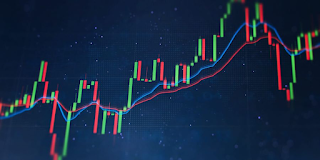# Exponential moving average indicatorExponential moving average indicator
Exponential moving average (EMA) is a kind of moving average which emphasizes the recent data points in the price chart of a security. Exponential moving average is also called weighted moving average. Exponential weighted average reacts more deeply to the recent market data compared to simple moving average.

## Calculating exponential moving average

Calculating the exponential moving average has one more step compared to the simple moving average. Imagine that you have chosen 20 periods for calculating the exponential moving average. In this case, you should remember to wait until the 20th day to calculate the simple moving average. On the 21st day, you can use yesterday’s simple moving average as the first exponential moving average.

Calculating SMA isn’t hard at all. In fact, the value of simple moving average is equal to the sum of closing prices of all candles divided by the number of periods. For example, the value of 20-day SMA is equal to the sum of closing prices of the recent 20 days divided by 20.

In the next step, the smoothing factor must be obtained to calculate the exponential moving average. The smoothing factor can be obtained via the following formula:

(the number of observations + 1) / 2

For example, this value for a 20-day SMA would be equal to 0.0952. Ultimately, the following formula must be used to calculate the exponential moving average:

EMA = closing price * (Smoothing + Yesterday’s EMA) * (Smoothing -1)

Exponential moving average is more sensitive to the recent data. Meanwhile, SMA treats all data equally. A short-term EMA is more sensitive to the recent data compared to a long-term EMA. For example, a 18.18% factor may be used for calculating the 10 period EMA, whereas the factor used for calculating the 20 period EMA is equal to 9.52%.

## What does EMA tell us?

12-day and 26-day exponential moving averages are pre-analyzed short-term indicators. 12-day and 26-day EMAs are used for calculating the Moving Average Convergence Divergence (MACD) and Percentage Price Oscillator (PPO). Generally, 50-day and 200-day EMAs are used for long-term analyses. When the price of a security crosses the 200-day EMA, this could be considered a reversing signal, if other technical indicators approve.

Traders who use technical analysis, use moving averages on a daily basis and consider them very useful and insightful. On the other hand, they know that if their analysis goes wrong, a tragic loss may be waiting for them. All moving averages which are used in technical analysis are considered lagging indicators according to their mathematical nature.

Therefore, when conducting technical analysis, a moving average must be used only to confirm the direction of the market or evaluate its momentum, because moving averages show trading opportunities when they are completely passed.

An exponential moving average reduces the lagging nature of moving averages. Since calculating EMA is more reliant on the recent market data, it has a closer relation to price action and reflects the changes more quickly. This could be beneficial when EMAs are used for finding trading opportunities.

Like any other technical indicator, EMA’s functionality is at maximum when used in popular markets. When the market is in a strong uptrend, the EMA also shows a rising trendline. This is also valid when facing a downtrend. A mindful trader pays attention to the EMA as much as he pays attention to the changes from one candlestick to the other (price action).

## Example of using EMA

EMAs are generally used for confirming other technical indicators. Exponential moving averages are more suitable for day traders and volatile markets. Most traders use EMAs to determine the overall direction of their transactions. If EMA shows a strong uptrend on the daily chart, a day trader may go long in this situation.

## Differences between EMA and SMA

The main difference between EMA and SMA is the excess sensitivity EMA has to recent market data. Let’s have a closer look. The exponential moving average is more sensitive to recent data, whereas simple moving averages treat all data equally. Both indicators are utilized by technical traders to obtain trading opportunities.

Since EMAs has more emphasize on the recent data, they are more sensitive to this groups of data. This will cause EMAs to be more accurate and this is the main reason why they are the first choice of many traders.

## Limitations of EMA

It isn’t yet determined if the recent data must weight more than the previous. Many traders believe that the recent data are better at reflecting the current status of the market. Meanwhile, other traders argue that being more sensitive to recent data may lead to false trading signals.

Generally, EMAs are reliant on both previous and recent market data. Many economists believe that markets are efficient. This means that the current price of a security is a reflection of all existing data right at this moment. If markets are really efficient, using previous data shouldn’t be able to tell us anything about the future movements of the market.

## Bottom line

A forex broker must provide its clients with such features and tools to ensure that they are analyzing the market carefully before starting to trade. Aron Groups Broker facilitates trading by providing its clients with MetaTrader 5 as a trading platform. In MetaTrader 5, traders can access numerous technical features including simple moving averages, exponential moving averages, momentum indicators, trendlines, etc. You can also open a demo account in Aron Groups Broker to test your strategy before starting to trade in the real market with your real assets.

Written by: Mohsen Mohseni (Aron Groups).